library(mlr3)
library(mlr3fairness)
library(mlr3learners)

## Why we need fairness visualizations:

Through fairness visualizations allow for first investigations into possible fairness problems in a dataset. In this vignette we will showcase some of the pre-built fairness visualization functions. All the methods showcased below can be used together with objects of type BenchmarkResult, ResampleResult and Prediction.

## The scenario

For this example, we use the adult_train dataset. Keep in mind all the datasets from mlr3fairness package already set protected attribute via the col_role “pta”, here the “sex” column.

t = tsk("adult_train")
t$col_roles$pta
#>  "sex"

We choose a random forest as well as a decision tree model in order to showcase differences in performances.

task = tsk("adult_train")$filter(1:5000) learner = lrn("classif.ranger", predict_type = "prob") learner$train(task)
predictions = learner$predict(tsk("adult_test")$filter(1:5000))

Note, that it is important to evaluate predictions on held-out data in order to obtain unbiased estimates of fairness and performance metrics. By inspecting the confusion matrix, we can get some first insights.

predictions$confusion #> truth #> response <=50K >50K #> <=50K 3499 485 #> >50K 261 755 We furthermore design a small experiment allowing us to compare a random forest (ranger) and a decision tree (rpart). The result, bmr is a BenchmarkResult that contains the trained models on each cross-validation split. design = benchmark_grid( tasks = tsk("adult_train")$filter(1:5000),
learners = lrns(c("classif.ranger", "classif.rpart"),
predict_type = "prob"),
resamplings = rsmps("cv", folds = 3)
)

bmr = benchmark(design)

## Fairness Prediction Density Plot

By inspecting the prediction density plot we can see the predicted probability for a given class split by the protected attribute, in this case "sex". Large differences in densities might hint at strong differences in the target between groups, either directly in the data or as a consequence of the modeling process. Note, that plotting densities for a Prediction requires a Task since information about protected attributes is not contained in the Prediction.

We can either plot the density with a Prediction

fairness_prediction_density(predictions, task)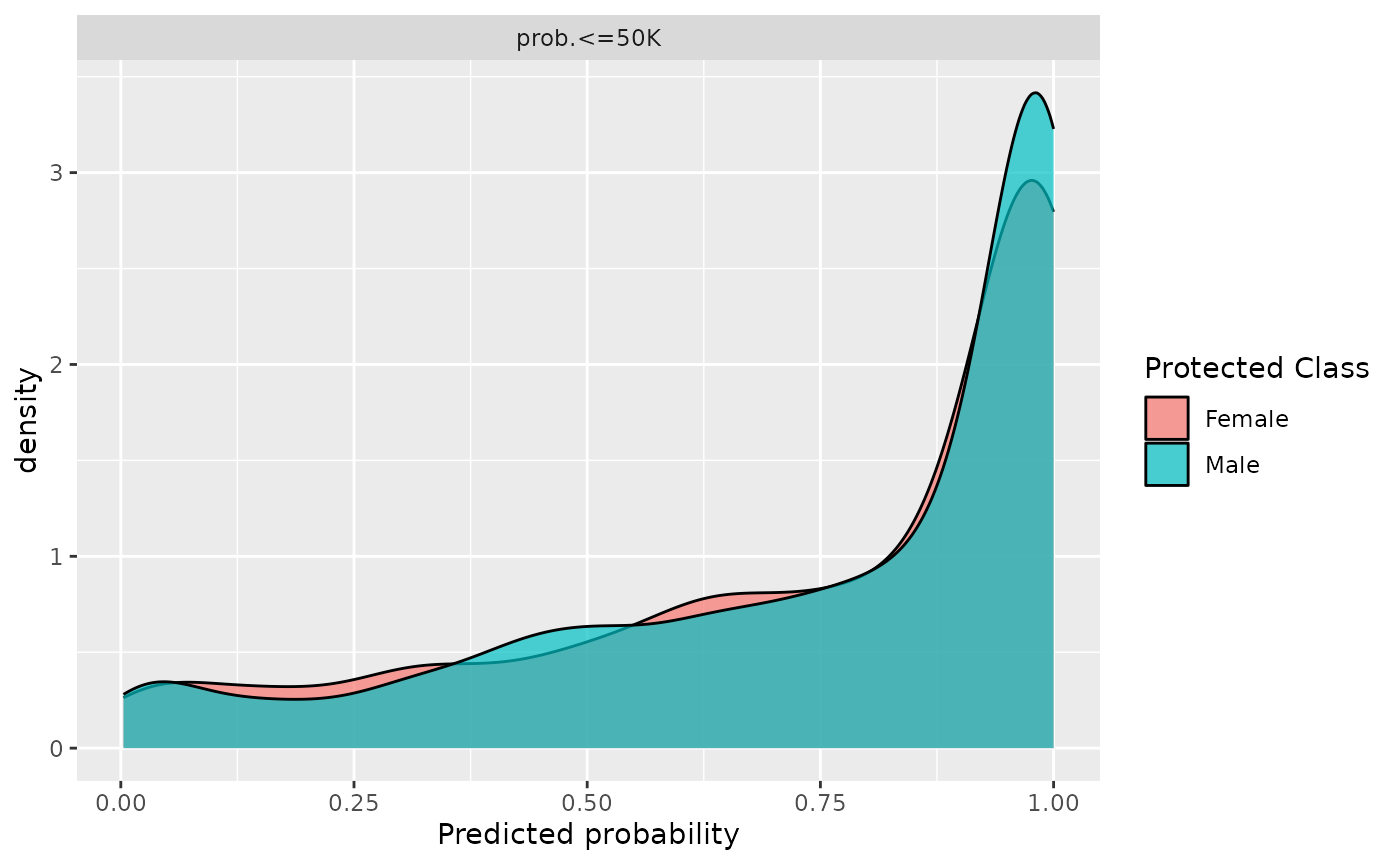or use it with a BenchmarkResult / ResampleResult:

fairness_prediction_density(bmr)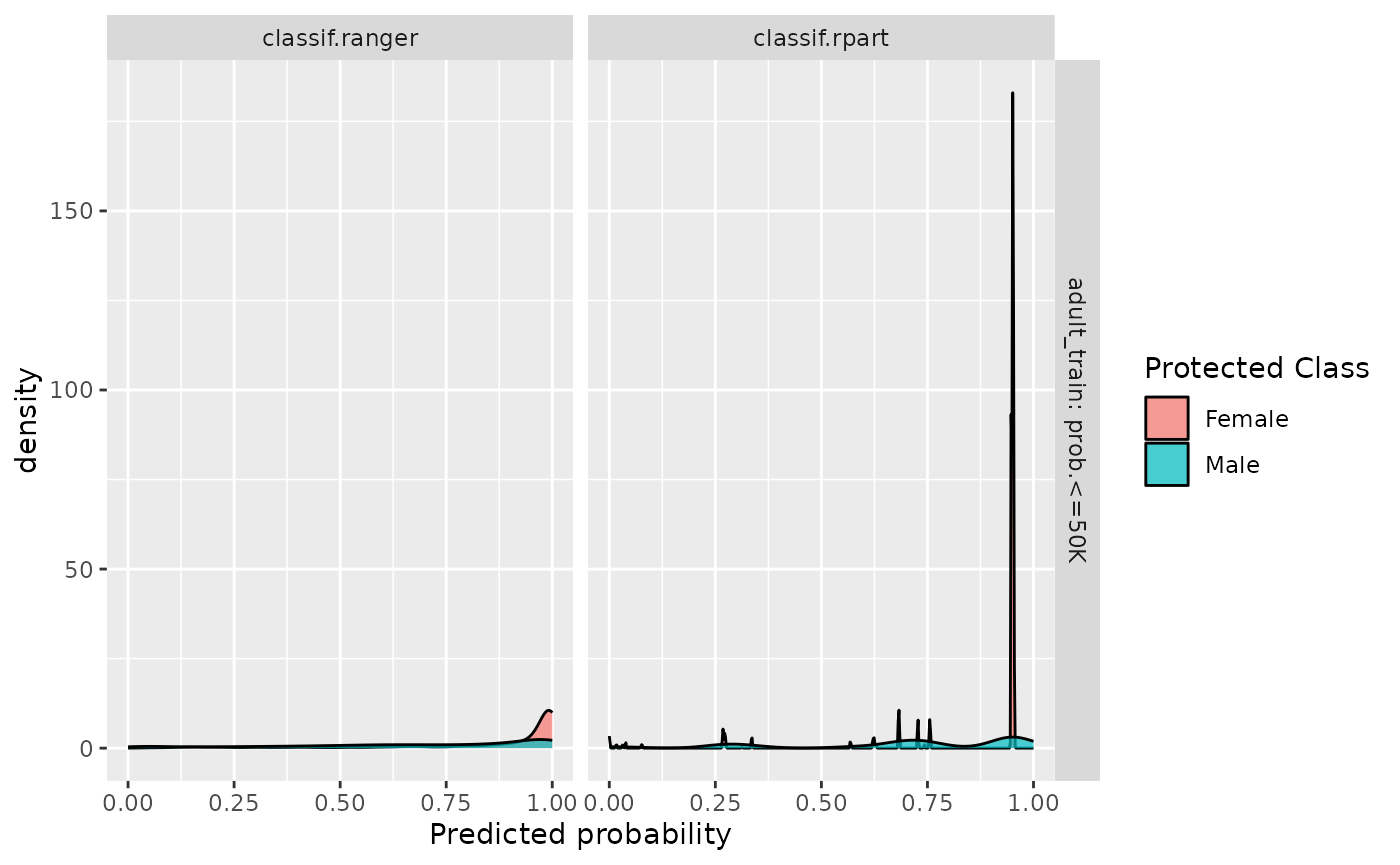In practice, we are most often interested in a trade-off between fairness metrics and a measure of utility such as accuracy. We showcase individual scores obtained in each cross-validation fold as well as the aggregate (mean) in order to additionally provide an indication in the variance of the performance estimates.

fairness_accuracy_tradeoff(bmr, msr("fairness.fpr"))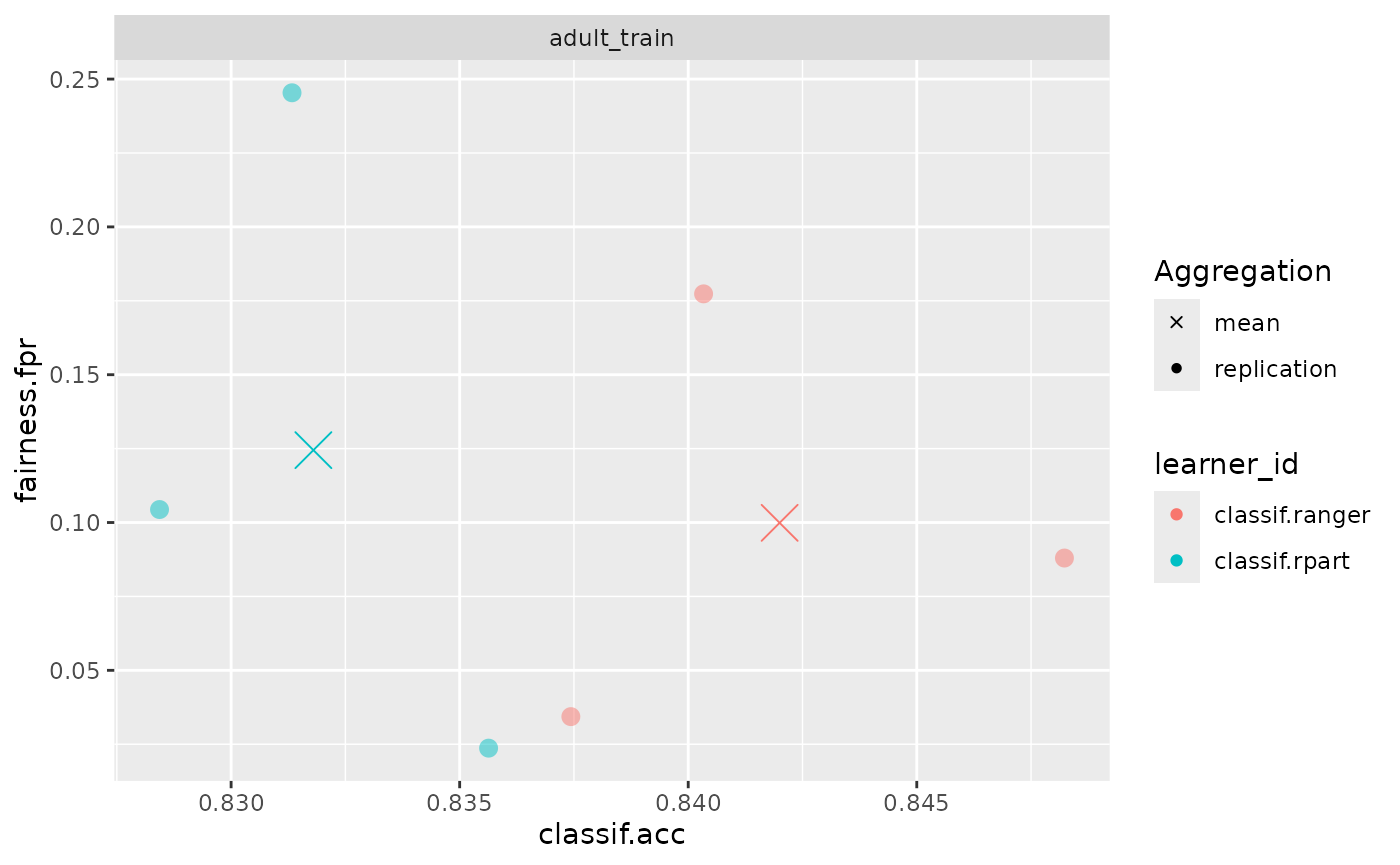## Fairness Comparison Plot

An additional comparison can be obtained using compare_metrics. It allows comparing Learners with respect to multiple metrics. Again, we can use it with a Prediction:

compare_metrics(predictions, msrs(c("fairness.fpr", "fairness.tpr")), task)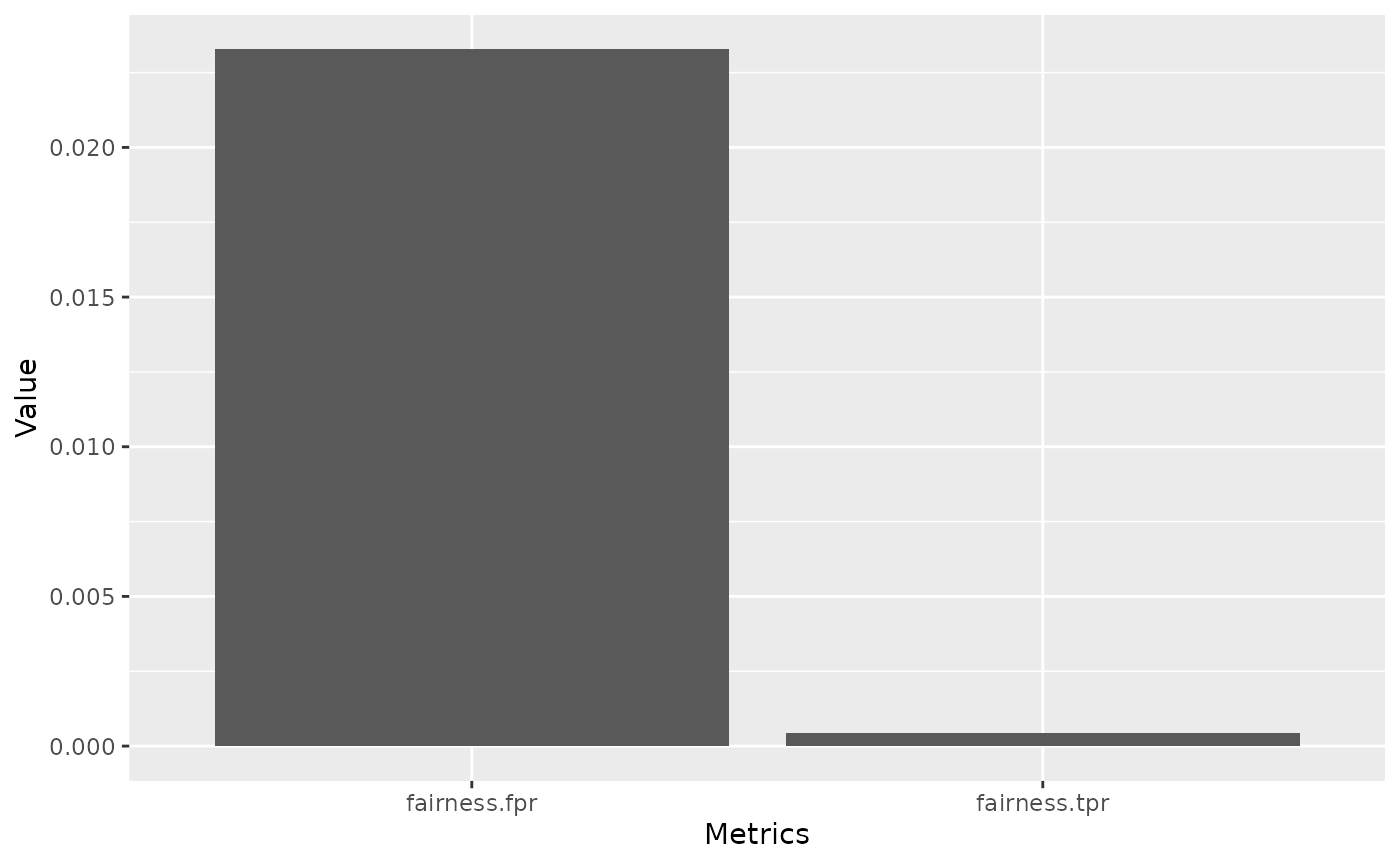or use it with a BenchmarkResult / ResampleResult:

compare_metrics(bmr, msrs(c("classif.ce", "fairness.fpr", "fairness.tpr")))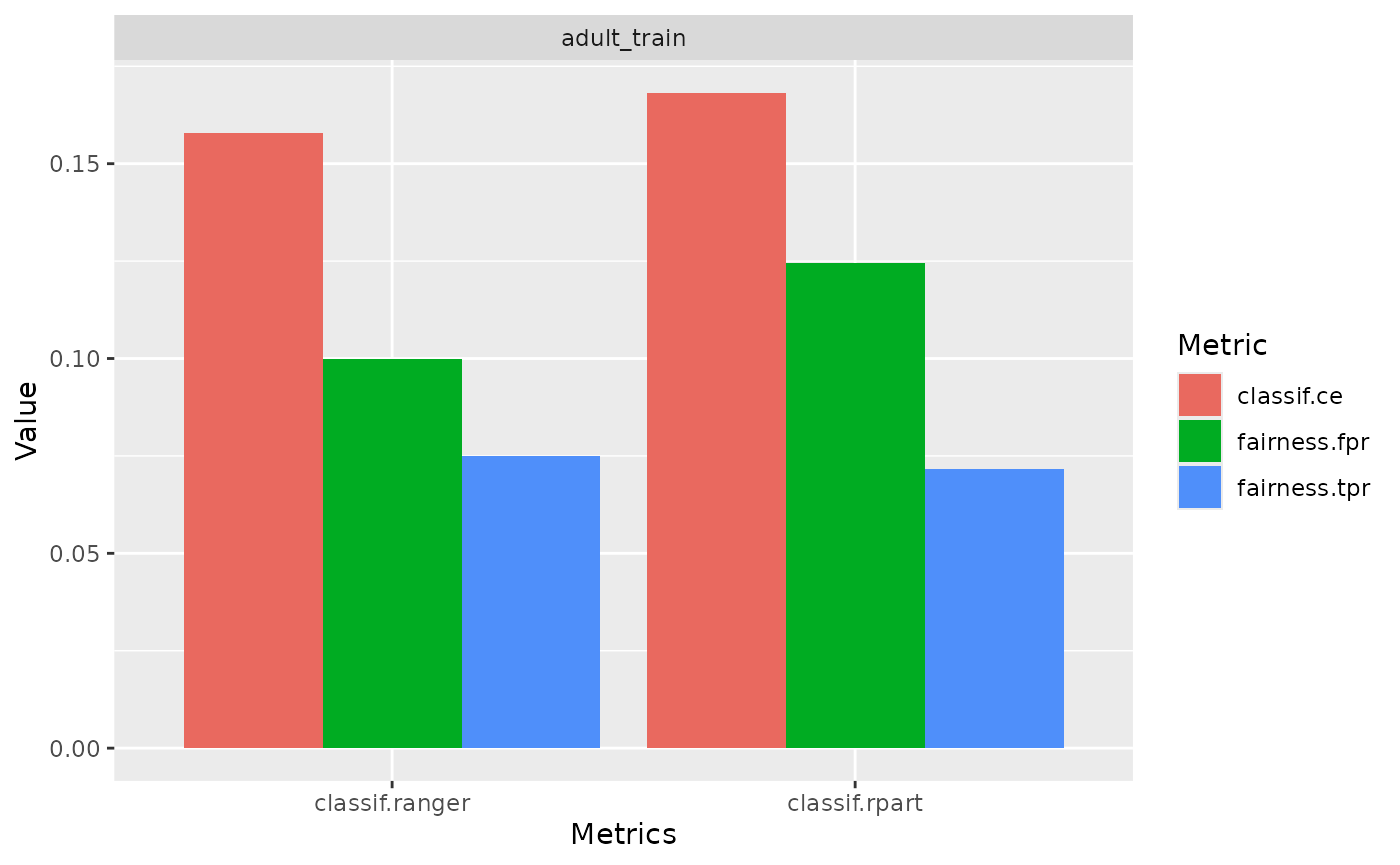## Custom visualizations

The required metrics to create custom visualizations can also be easily computed using the $score() method. bmr$score(msr("fairness.tpr"))
#>    nr     task_id     learner_id resampling_id iteration fairness.tpr
#> 1:  1 adult_train classif.ranger            cv         1   0.05835514
#> 2:  1 adult_train classif.ranger            cv         2   0.10494351
#> 3:  1 adult_train classif.ranger            cv         3   0.06321038
#> 4:  2 adult_train  classif.rpart            cv         1   0.04899263
#> 5:  2 adult_train  classif.rpart            cv         2   0.07506810
#> 6:  2 adult_train  classif.rpart            cv         3   0.05293768
#> Hidden columns: uhash, task, learner, resampling, prediction

## Interpretability

Fairness metrics, in combination with tools from interpretable machine learning can help pinpointing sources of bias. In the following example, we try to figure out which variables have a high feature importance for the difference in classif.eod, the equalized odds difference. In the following example

set.seed(432L)
library("iml")
library("mlr3fairness")

learner = lrn("classif.rpart", predict_type = "prob")
task$filter(sample(task$row_ids, 2000))
task$select(c("sex", "relationship", "race", "capital_loss", "age", "education")) target = task$target_names
learner$train(task) model = Predictor$new(model = learner,
data = task$data()[,.SD, .SDcols = !target], y = task$data()[, ..target])

custom_metric = function(actual, predicted) {
compute_metrics(
data = task$data(), target = task$target_names,
protected_attribute = task$col_roles$pta,
prediction = predicted,
metrics = msr("fairness.eod")
)
}

imp <- FeatureImp$new(model, loss = custom_metric, n.repetitions = 5L) plot(imp)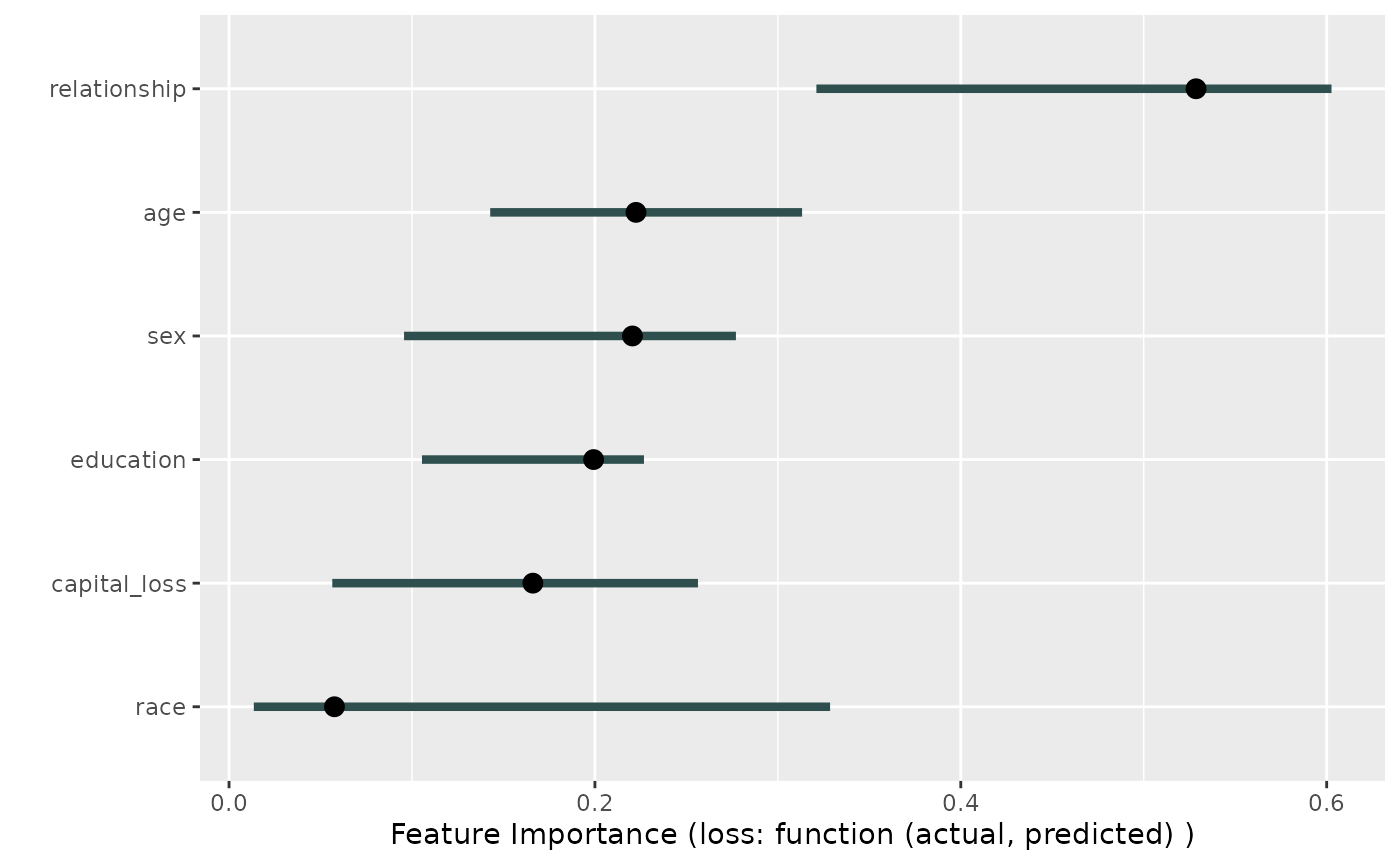We can now investigate this variable a little deeper by looking at the distribution of labels in each of the two groups. data = task$data()
data[, setNames(as.list(summary(relationship)/.N),levels(data\$relationship)), by = "sex"]
#>       sex  Husband Not-in-family Other-relative Own-child  Unmarried      Wife
#> 1:   Male 0.611516     0.2048105     0.02332362 0.1231778 0.03717201 0.0000000
#> 2: Female 0.000000     0.3662420     0.03980892 0.1671975 0.26910828 0.1576433

We can see, that the different levels are skewed across groups, e.g. 25% of females in our data are unmarried in contrast to 3% of males are unmarried.